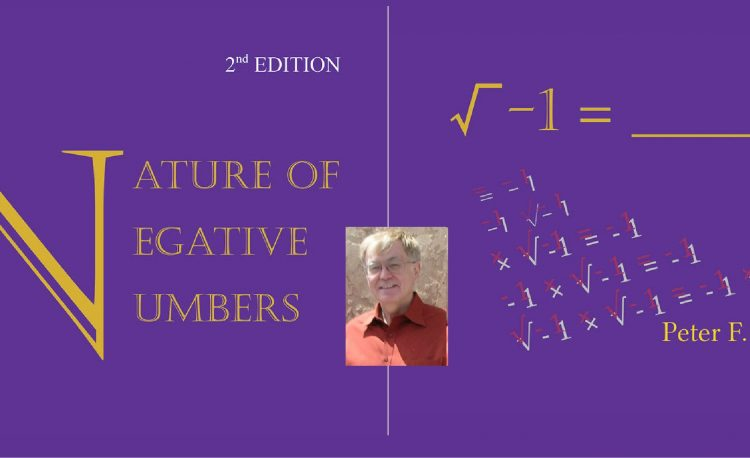# The Veritable Number System

It seems quite outrageous to think that our wonderfully useful and familiar “real number system” in mathematics is flawed. How can that be? But if you think for a moment, there are two that come to mind pretty quickly:

• dividing by zero
• the square root of negative numbers

But what you may not know is that the concept of negative numbers really doesn’t exist in the physical universe. It comes from our mathematical systems, not from nature.

But thanks to critical thinker and mathematician Peter Erickson, we have an alternative that plugs the holes, and rights the wrong with our real number system and replaces it with what he calls the “Veritable Number System”.

### The Nature of Negative Numbers

What is the big deal with the square root of negative one? Well, the problem is, in our real number system, is that if you multiply two negative numbers together, you always get a positive number. The definition of square root, is that the answer is a number that when multiplied by itself, returns that number.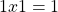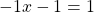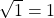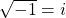What happens with the square root of negative one? In our real number system, they call that an “imaginary” number or the letter “i”. Think about that: “imaginary” means not real. It violates our “real” number system. Yet, we allow our teachers to teach us this without questioning. That same theme that runs through the history of teaching where we are not told how to find truth, but are simply told the truth. Questioning it is not allowed.

So what Erickson says in his book is quite simple: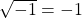We need to redefine square root to mean that the square root of a negative number, will be a negative number.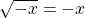It turns out that in our numbering system, the square root sign has significance. Erickson is stating that it’s significance needs to change. This is a much saner solution than creating an ad-hoc “imaginary” symbol “i”.

### Negative Numbers in Physics

During his lecture on his book in 2017 at the University of British Columbia in Vancouver Canada, Peter told me he has been coming to our conferences to talk about his work in the hopes that it would help those working on the cutting edge of physics. Peter has in fact been presenting talks on math for well over 10 years to the CNPS (formally the NPA).

It turns out that my father, Robert de Hilster, has been working on describing what is happening physically inside electronic components according to our particle model and my father actually stated to me that negative numbers don’t and can’t exist in the physical world. It is an artificial construct of our mathematical system.

One simple example of this is the sine curve. Normally, the sine or cosine curves include negative values because we center the curve at zero on the x-axis. Sine curve-like behaviors happen in electronic circuits when circuits are turned on and current flow builds up and falls off.

Even though electrical engineering often shows this as a sinusoidal wave with the curve going negative, my father pushes this curve up so that the bottom of the curve touches zero. The curve now shows the number of particles flowing in a circuit.